# OECD Tourism flows data analysis 1 - Load CSV data into R.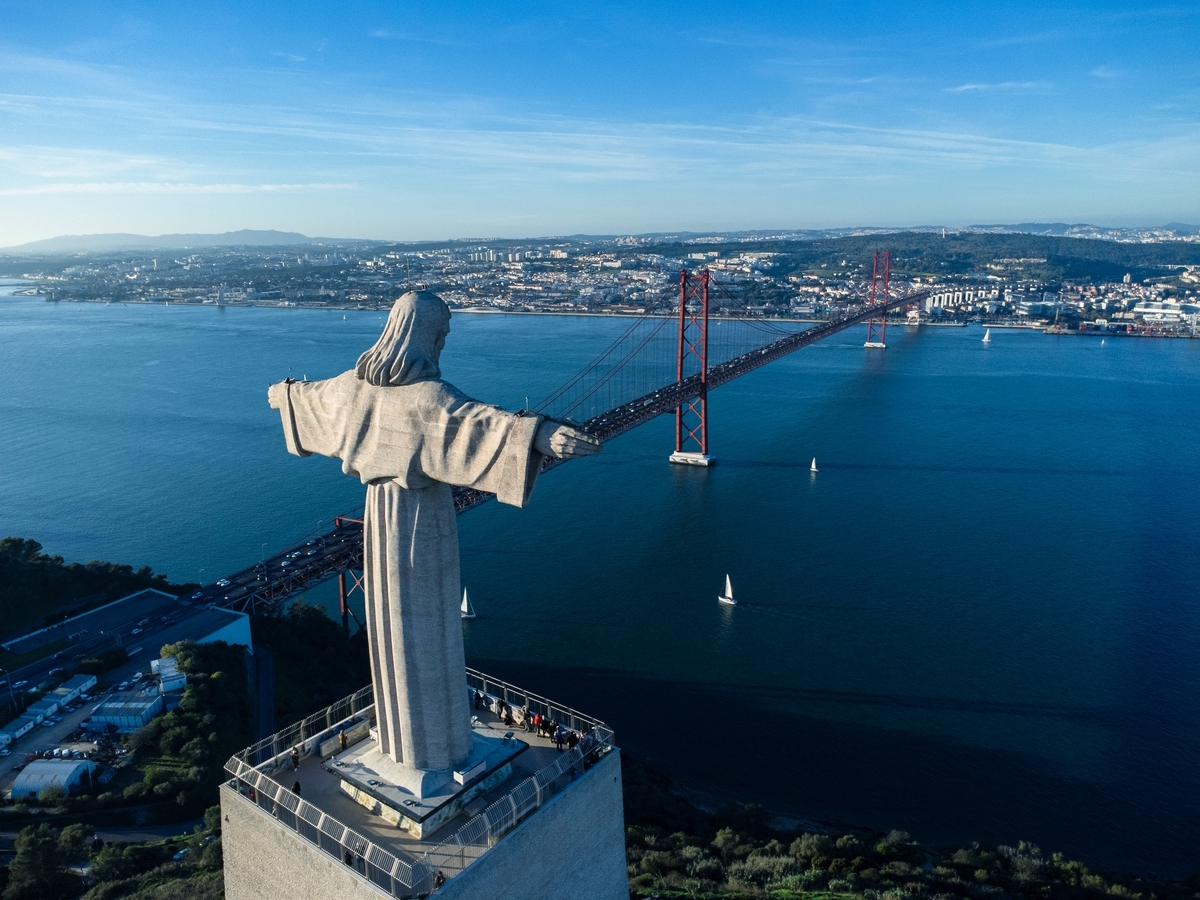UnsplashErik Knoefが撮影した写真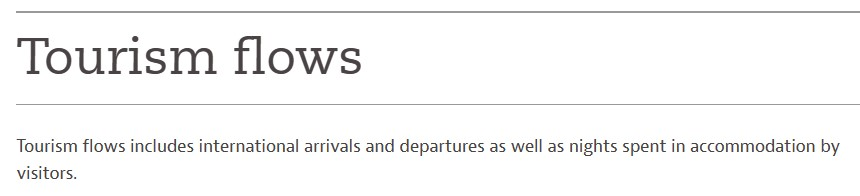In this post, I will analyze OECD Tourism flows data analysis.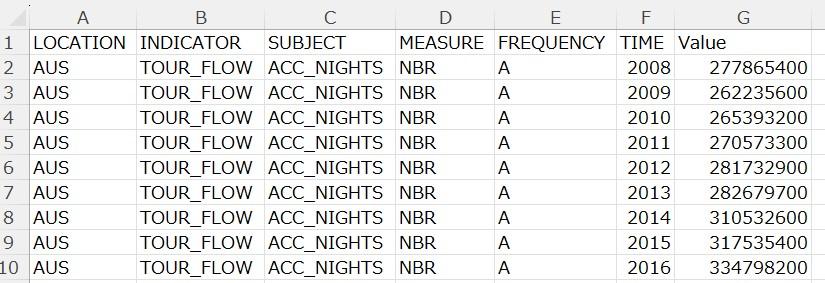I also downladed GDP per capita data from OECD web site.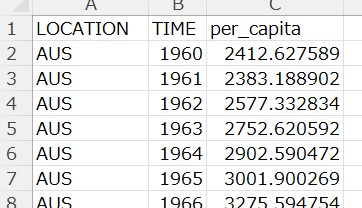I use R for data analysis.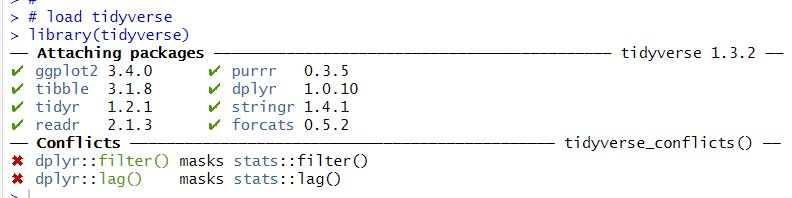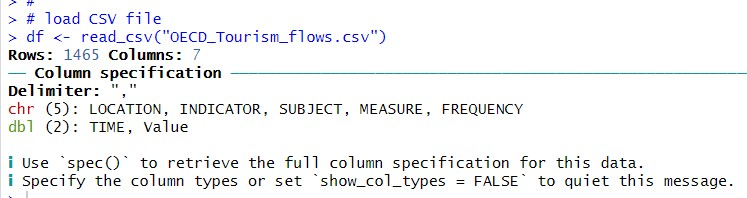I also load GDP per capita CSV file.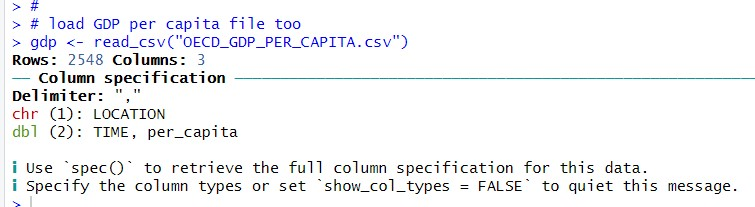Then, I merge 'df' and 'gdp' with inner_join() function and make a new data frame.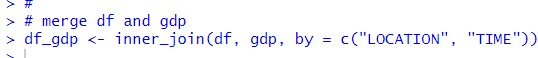Let's use summary() function to get 'df_gdp' summary statistics.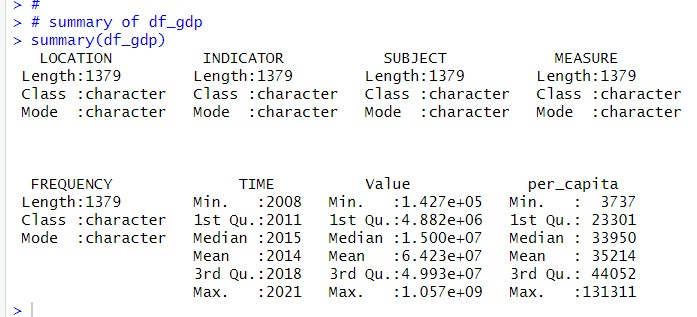From 'LOCATION' to 'FREQUENCY', there is not so much information, I just know there are 1379 observations.

For TIME, I know it strats from 2008 to 2921.
Value comes from 'df' and 'per_capita' comes from 'gdp'.

Let's check LOCATION with table() function.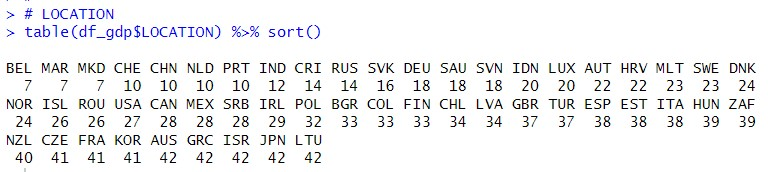LOCATIONs which have most observations are AUS, GRC, ISR, JPN and LTU.

Let's see INDICATOR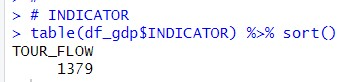INDICATOR has only one value, TOUR_FLOW, so I can remove this variable.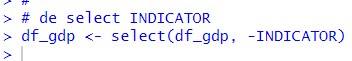Let's check SUBJECT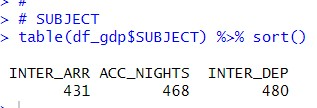INTER_ARR is arrival number of tourist, ACC_NIGHTS is accomodation nights and INTER_DEP is departure number of tourist. I keep SUBJECT.

Let's check MEASURE.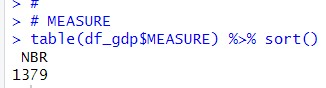MEASURE has only one value, NBR. so I remove MEASURE from df_gdp.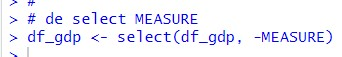Let's check FRQUENCY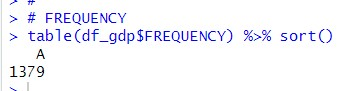FREQUENCY has only one value, A. So I can remove FREQUENCY.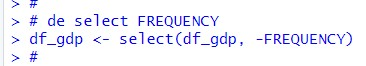Then, I mutate LOCATION and SUBJECT to factor class.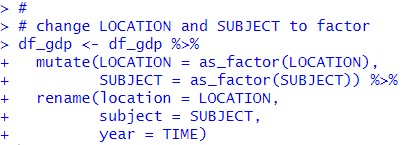I also changed variavle names.

All right, let's use summary() function again.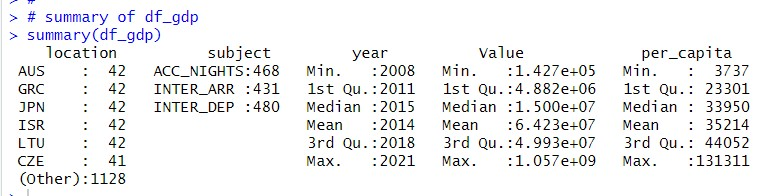I think Value can be re-scale. I divide Value by 10000.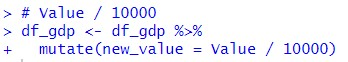Let's see summary.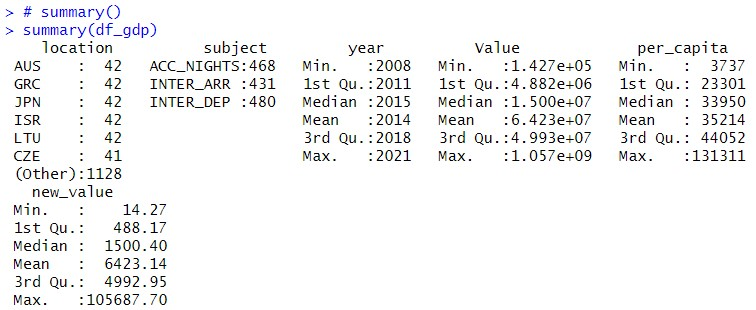That's it for this post. I will continure analysis in the next post. Thank you!

Next post is